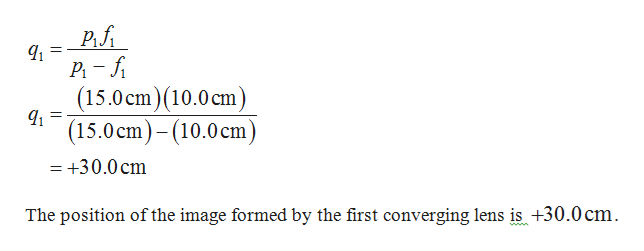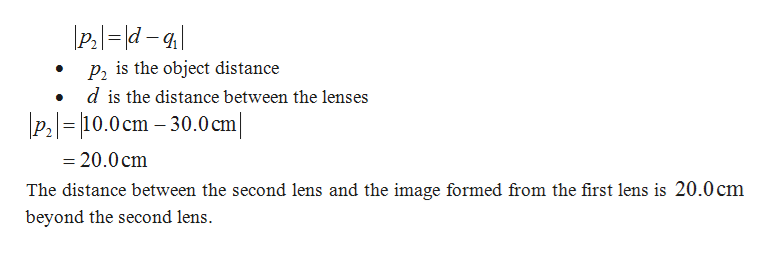# An object is placed15.0 cm from a first converginglens of focal length 10.0 cm. A second converging lens withfocal length 5.00 cm is placed 10.0 cm to the right of the firstconverging lens. (a) Find the position q1 of the image formedby the first converging lens. (b) How far from the second lensis the image of the first lens? (c) What is the value of p 2, theobject position for the second lens? (d) Find the position q 2 ofthe image formed by the second lens. (e) Calculate the magnificationof the first lens. (f) Calculate the magnification of thesecond lens. (g) What is the total magnification for the system?(h) Is the final image real or virtual? Is it upright or inverted(compared to the original object for the lens system)?

Question
1 views

An object is placed
15.0 cm from a first converging
lens of focal length 10.0 cm. A second converging lens with
focal length 5.00 cm is placed 10.0 cm to the right of the first
converging lens. (a) Find the position q1 of the image formed
by the first converging lens. (b) How far from the second lens
is the image of the first lens? (c) What is the value of p 2, the
object position for the second lens? (d) Find the position q 2 of
the image formed by the second lens. (e) Calculate the magnification
of the first lens. (f) Calculate the magnification of the
second lens. (g) What is the total magnification for the system?
(h) Is the final image real or virtual? Is it upright or inverted
(compared to the original object for the lens system)?

check_circle

Step 1

a). Formula to calculate the image distance is,help_outlineImage TranscriptioncloseP,fi P1 – fi (15.0 cm)(10.0 cm) (15.0 cm)- (10.0cm) = +30.0 cm The position of the image formed by the first converging lens is +30.0 cm. fullscreen
Step 2

b).

Formula to calculate the o...help_outlineImage Transcriptionclose\P:|= |d - q| P2 is the object distance d is the distance between the lenses \P3|= |10.0 cm – 30.0 cm| %3D %3D = 20.0 cm The distance between the second lens and the image formed from the first lens is 20.0 cm beyond the second lens. fullscreen

### Want to see the full answer?

See Solution

#### Want to see this answer and more?

Solutions are written by subject experts who are available 24/7. Questions are typically answered within 1 hour.*

See Solution
*Response times may vary by subject and question.
Tagged in

### Ray Optics